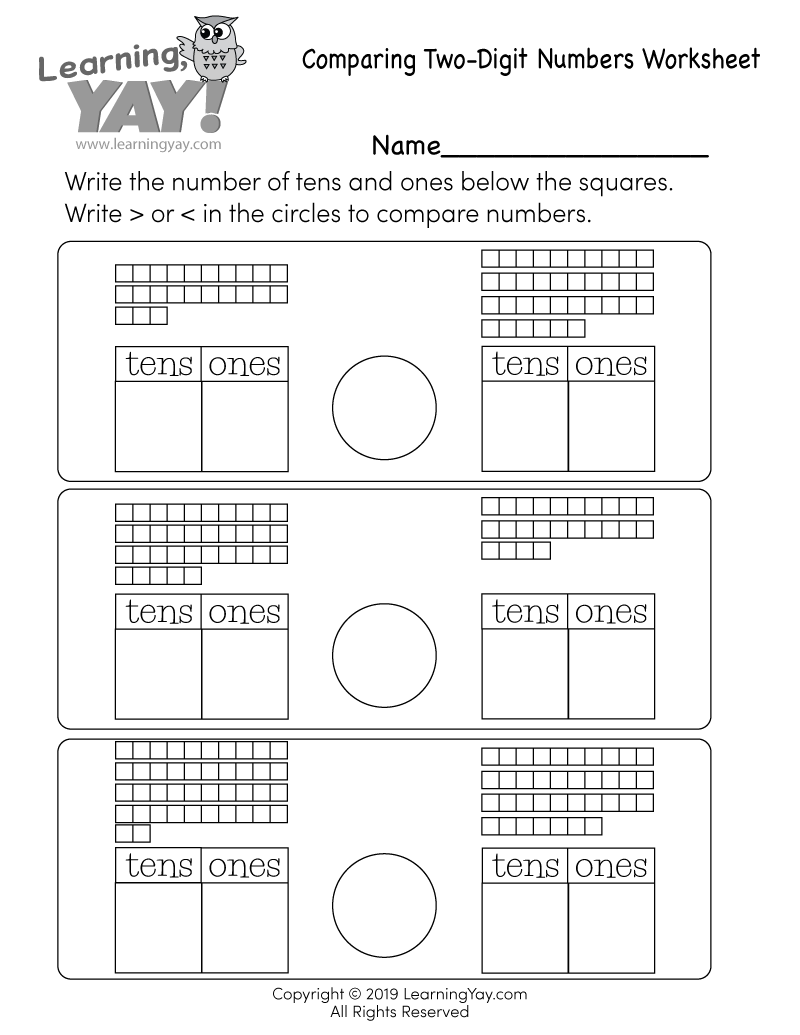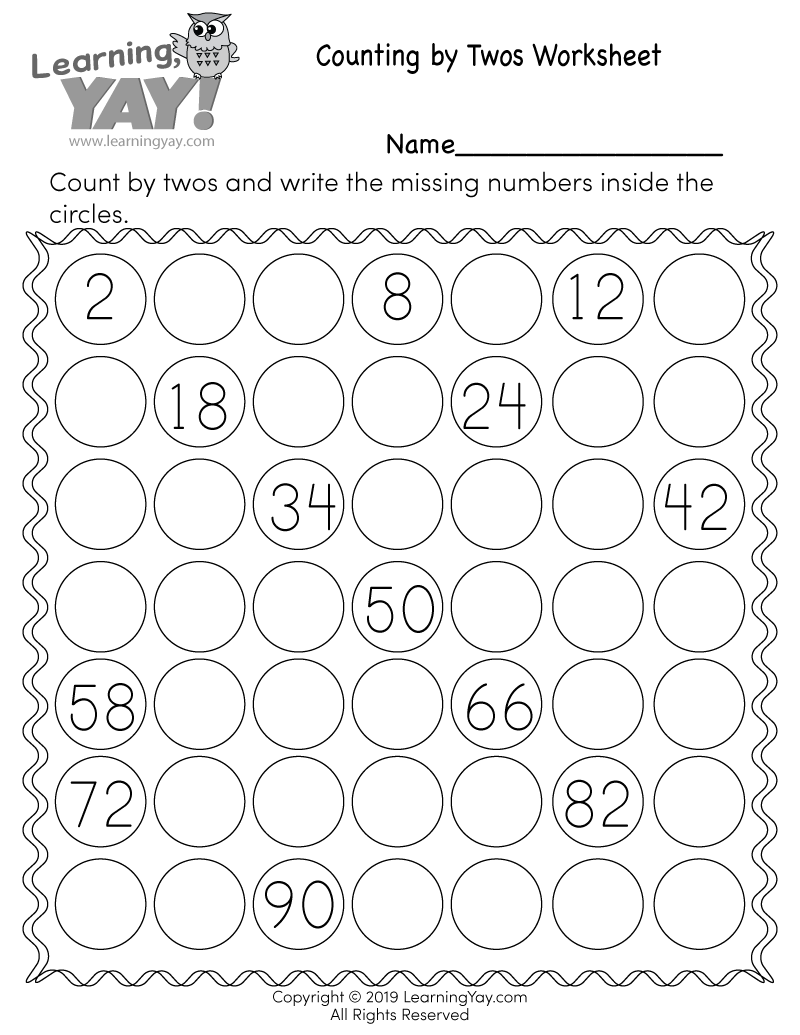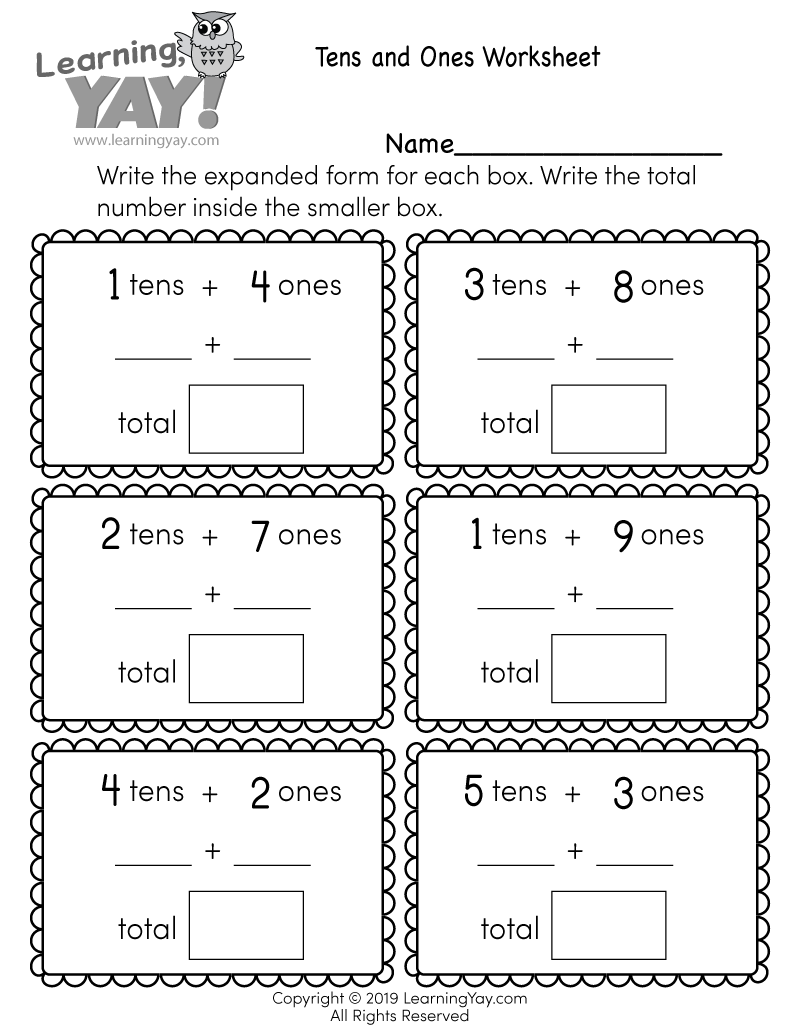# Comparing Two-Digit Numbers Worksheet

This free number sense worksheet can help first graders improve their understanding of place value and the properties of operations by comparing two two-digit numbers based on meanings of the tens and ones digits. Students are asked to write the number of tens and ones below the squares in order to compare each two-digit number using a greater than or less than symbol.This free printable math worksheet is aligned to first grade Common Core standards. It is an excellent resource for teachers and parents who are teaching the following standards.
##### 1.NBT.B.2 - Common Core ID

Understand that the two digits of a two-digit number represent amounts of tens and ones. Understand the following as special cases:

##### 1.NBT.B.2a - Common Core ID

10 can be thought of as a bundle of ten ones — called a “ten.”

##### 1.NBT.B.3 - Common Core ID

Compare two two-digit numbers based on meanings of the tens and ones digits, recording the results of comparisons with the symbols >, =, and <. Use place value understanding and properties of operations to add and subtract.

Common Core » 1st Grade Math Standards » Number & Operations in Base Ten » Understand place value. » 1.NBT.B.2, 1.NBT.B.2a, 1.NBT.B.3

## There are multiple ways to get this worksheet.

### You might also like these math worksheets: